Back to Kemp Acoustics HomeNext: Numerical implementation of multimodal Up: Multimodal radiation impedance of Previous: Analysis   Contents

## Results

Taking
gives the radiation impedance of a square duct terminated in an infinite baffle. It is interesting to compare this with the result derived by Zorumski  for the radiation impedance for a circular duct terminated in an infinite baffle. The direct impedance of the plane wave mode (
,
) for a square duct of half widthis shown in figure 3.5(a). Also shown is the equivalent for a circular duct of the same cross-sectional area (radius
). The results show very similar behaviour.

Figure 3.5(b) shows the impedance of the plane wave pressure mode () coupled with the
velocity mode for a square duct of half widthalong with the plane wave pressure mode coupled with the pressure mode with two nodal circles in a cylindrical duct of the same cross-sectional area. While the analogue between the two situations is less strong, the same qualitative behaviour is observed.

Figures 3.6(a) and 3.6(b) display various direct radiation impedances for a square duct. As was the case with circular cross-section, the radiation impedance starts at zero for the zero frequency limit (as for the ideal open end condition). At low frequencies the impedance has a small, positive imaginary value. As with the circular cross-section discussion, this means that the acoustic pressure has a node a small distance from the end of the tube due to out of phase reflection of sound. At high frequencies the impedance converges on the infinite cylindrical pipe termination value of 1 (or
before normalisation). Modes with shorter transverse wavelengths converge more slowly.

Figure 3.7(a) and 3.7(b) display coupled radiation impedances for a square duct. Figure 3.7(a) shows examples where the pressure and velocity are direct in one dimension and coupled in the other. Figure 3.7(b) shows examples where the pressure and velocity are coupled in both dimensions with a correspondingly smaller range of impedance values. As with the cylindrical geometry, the coupled radiation impedance and therefore the amount of inter-modal coupling tends to zero in both the zero frequency and high frequency limits.

Figure 3.8(a) shows the effect of varying the aspect ratio (
) on the plane wave pressure and plane wave velocity radiation impedance. This graph is in agreement with the values of the rectangular piston radiation impedance as published by Burnett and Soroka . Making the opening rectangular rather than square while keeping the cross-sectional area constant is observed to make the direct impedance of the plane mode converge much more slowly on the characteristic impedance termination value. Physically this is a consequence of the opening having one very narrow dimension, meaning that higher frequencies must be accessed before the effects of diffraction at the opening disappear. The direct impedance of the plane mode in a duct of a given aspect ratio
will equal that of duct of aspect ratioby symmetry. This effect only holds if the pressure distribution has the same number of nodal lines in both theand
directions (ie.) and the velocity distribution similarly has
.

Figure 3.8(b) shows the effect of aspect ratio on a coupled impedance. The velocity distribution has twice as many nodes on theaxis as there are on theaxis while the pressure mode is planar. The
case shows the coupled impedance for a square duct. Setting
, the duct width alongdirection is half that along. The transverse wavelength of the velocity distribution is therefore four times as large alongas along. The wavelength along one dimension is then very short, and we observe that higher frequencies must be reached before coupling takes place for the rectangular duct in comparison to a square duct of the same area. Forthe duct is twice as wide in thedirection meaning that the transverse wavelength is the same alongas along. In this this case we therefore observe that coupling with the plane pressure mode can happen at lower frequencies for the rectangular duct.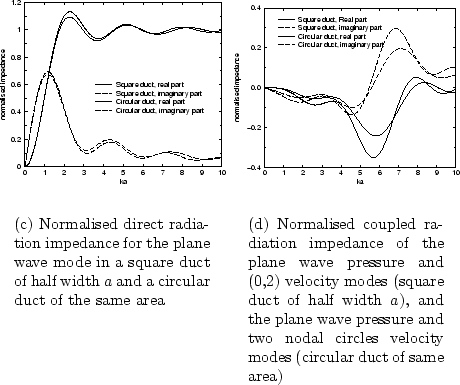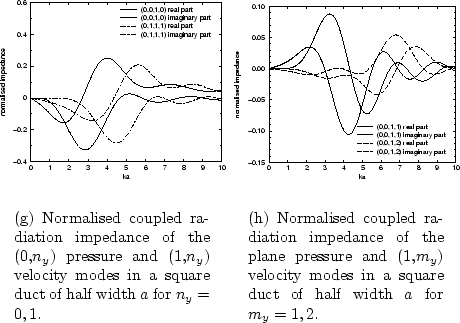Back to Kemp Acoustics HomeNext: Numerical implementation of multimodal Up: Multimodal radiation impedance of Previous: Analysis   Contents
Jonathan Kemp 2003-03-24